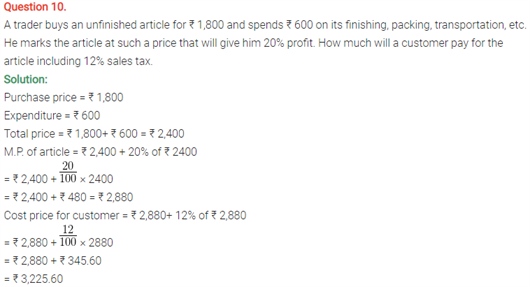# Selina Concise Maths Solution for ICSE Class 10 Chapter 1 Value Added Tax

## Selina Class 10 Maths Solutions for Chapter 1

ICSE Solutions For Class 10 Maths are arranged in a systematic manner according to each question and will definitely ensure that you grasp the concepts in a much effective manner. These solutions comprise of all the important concepts and formulas taught in the class along with all examples which will definitely help you remember important topics while preparing for maths exams. Tax on the rate of goods and services is a very important concept to understand the logic behind the prices of goods. With these ICSE Class 10 Maths Selina solutions, you will understand this chapter in detail.

Get the ICSE Solutions Of Class 10 Maths Chapter 1 Value Added tax Below:

### Selina class 10 Maths solutions for chapter 1, question 1 and 2: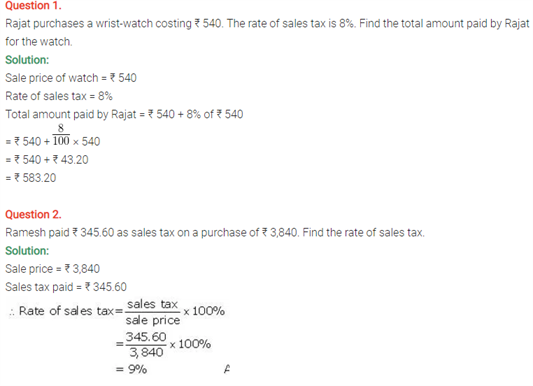### Selina class 10 Maths solutions for chapter 1, question 3 and 4 :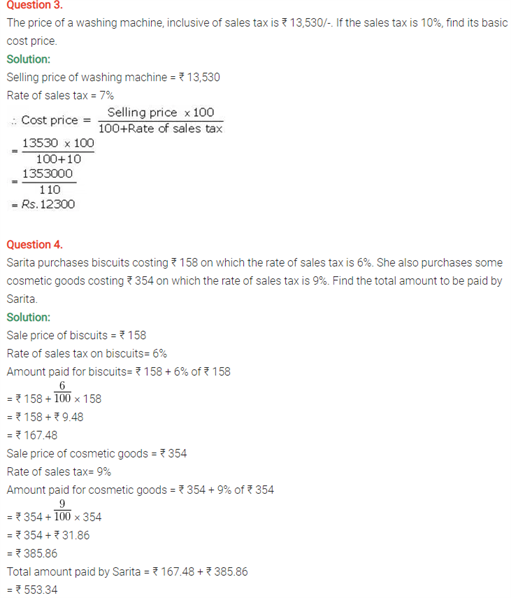### Selina class 10 Maths solutions for chapter 1, question 5  :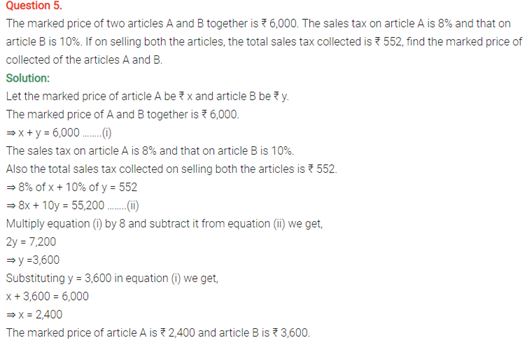### Selina class 10 Maths solutions for chapter 1, question 6 :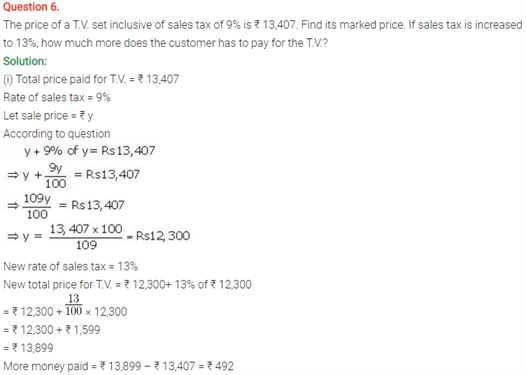### Selina class 10 Maths solutions for chapter 1, question 7 and 8 :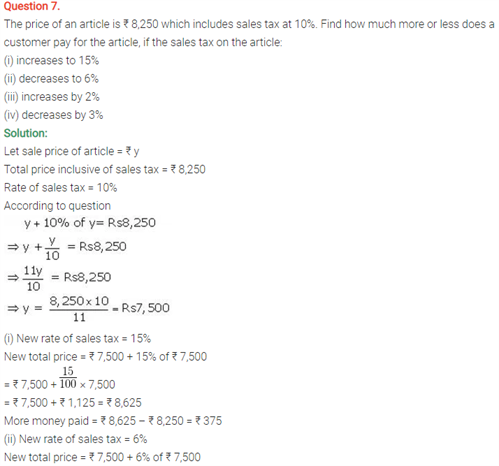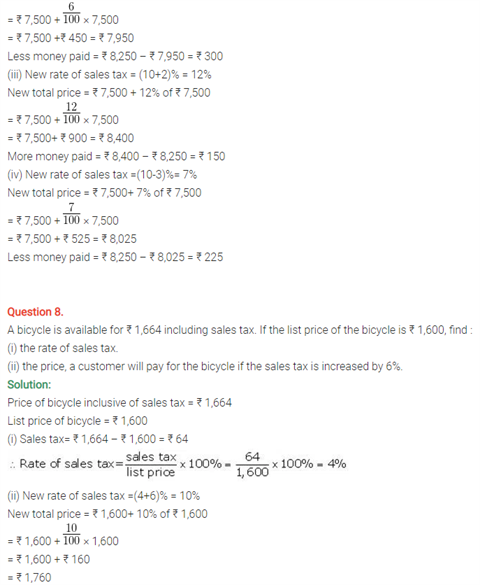### Selina class 10 Maths solutions for chapter 1, question 9 :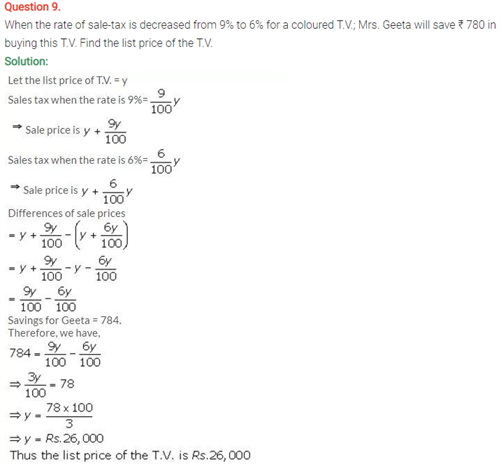### Selina class 10 Maths solutions for chapter 1, question 10 :